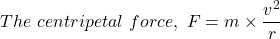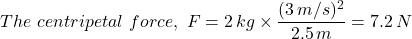calculate the tension in a horizontal string that whirls a 2kg toy in a circle of radius 2.5 meters when it moves 3m/s on icy surface​

Question

calculate the tension in a horizontal string that whirls a 2kg toy in a circle of radius 2.5 meters when it moves 3m/s on icy surface​

in progress 0
5 months 2021-08-26T01:21:36+00:00 1 Answers 3 views 0

The tension in the horizontal string is 7.2 N

Explanation:

With the assumption that the ice surface has no friction, the tension in the horizontal string is equal to the centripetal force, ‘F’, acting on the string to keep it in circular motionWhere;

m = The mass of the object in the circular

v = The tangential velocity of the object

r= The radius of the circle through whose path the object is moved

The given parameters are;

The mass of the toy that is whirled in a circle by the string, m = 2 kg

The radius of the circle the toy’s path turns, r = 2.5 meters

The velocity of the toy at the instant the tension is sought, v = 3 m/s

Therefore, we get;The centripetal force, F = 7.2 N = The tension in the horizontal string

∴ The tension in the horizontal string = 7.2 N.